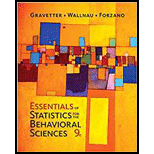Chapter 10.3, Problem 1LC### Essentials of Statistics for The B...

9th Edition
Frederick J Gravetter + 2 others
ISBN: 9781337098120

#### Solutions

Chapter
Section### Essentials of Statistics for The B...

9th Edition
Frederick J Gravetter + 2 others
ISBN: 9781337098120
Textbook Problem

# What is the value of the independent-measures t statistic for a study with n = 10 participants in each treatment if the data produce M = 38 and SS = 200 for the first treatment and M = 33 and SS = 160 for the second treatment? a. t = 1.25 b. t = 2.50 c. t = 0.23 d. t = 5 20 = 1.12

To determine

To find: The independent-measures t-statistics for the given question.

Explanation

Given info:

Two samples with same sample size 10 are used to evaluate the mean difference between the two populations. The sample mean and sum of squared difference for the first sample is M1=38 and SS1=200 . For the second sample the values are M2=33 and SS2=160

Reason for correct answer:

Calculations:

The degrees of freedom for two samples is:

df1=n11=9

Similarly,

df2=n21=9

The pooled variance, sp2 , is calculated using formula:

sp2=SS1+SS2df1+df2

Substitute 200 for SS1 , 160 for SS2 and 9 for both df1 and df2 in the above formula

sp2=200+1609+9=36018=20

The formula for estimated standard error of the difference is

sM1M2=<

### Still sussing out bartleby?

Check out a sample textbook solution.

See a sample solution

#### The Solution to Your Study Problems

Bartleby provides explanations to thousands of textbook problems written by our experts, many with advanced degrees!

Get Started

## Additional Math Solutions

#### Find more solutions based on key concepts